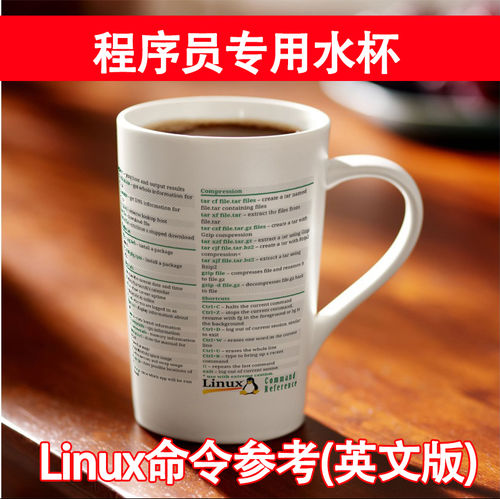### 新的 Range

``````func doSomething(with range: Range) {
for number in range {
...
}
}

doSomething(with: 0..<10)
doSomething(with:="" 0...10)=""``````

``````func doSomething(for range: IterableRange)
where IterableRange: Sequence, IterableRange.Iterator.Element == Int {
for number in range {
...
}
}

doSomething(with: 0..<10)
doSomething(with:="" 0...10)=""``````

### 元组转换

``````typealias Tuple = (foo: Int, bar: Int)

let dict: [Int: Int] = [1: 100, 2: 200]

for tuple: Tuple in dict {
print("foo is \(tuple.foo) and bar is \(tuple.bar)")
}``````

``````for tuple in dict {
let tuple: Tuple = (foo: tuple.key, bar: tuple.value)
print("foo is \(tuple.foo) and bar is \(tuple.bar)")
}``````

### 最后的想法